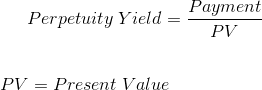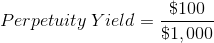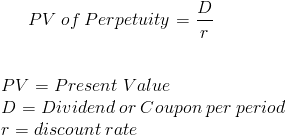# Perpetuity YieldThe formula that is used to calculate the yield on a perpetuity is the payment divided by the present value of the perpetuity. A perpetuity is a form of annuity that has an infinite amount of periodic payments.

A few examples of when the perpetuity yield formula may be used, is to evaluate consols in the UK, preferred stocks, and stocks that have zero growth. Typically, investments such as preferred stock and stocks that aren't expected to grow are valued for their ongoing dividends and not based on appreciation. Theoretically, this ongoing dividend is treated like a perpetuity. However, the rate may change over time which will affect the value of the perpetual investment.

## Example of the Perpetuity Yield Formula

An example of the perpetuity yield formula would be to assume an investor is reviewing an investment that is priced at \$1000 and pays \$100 in annual payments. Using the formula at the top of the page, this can be shown as:This results in a rate of .10, or 10%. Keep in mind that this rate does not necessarily look at risk. If this company is not around after a few years, the investment isn't really a perpetuity, now looking at it in hindsight. Perpetuity formulas tend to be conceptual and not certain. An investor will want a higher rate of return for a riskier investment.

## How is the Perpetuity Yield Formula derived?

To find the formula for the perpetuity yield, we first look at the formula for the present value on perpetuities:In order to isolate the rate, both sides can be multiplied by r/PV. This results in the formula that can be seen at the top of the page.

New to Finance?